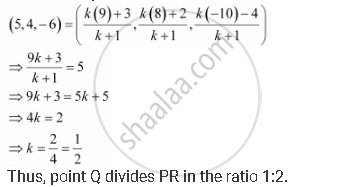CBSE (Arts) Class 11CBSE
Share

# Given that P (3, 2, –4), Q (5, 4, –6) and R (9, 8, –10) Are Collinear. Find the Ratio in Which Q Divides Pr. - CBSE (Arts) Class 11 - Mathematics

ConceptThree - Dimensional Geometry Section Formula

#### Question

Given that P (3, 2, –4), Q (5, 4, –6) and R (9, 8, –10) are collinear. Find the ratio in which Q divides PR.

#### Solution

Let point Q (5, 4, –6) divide the line segment joining points P (3, 2, –4) and R (9, 8, –10) in the ratio k:1.

Therefore, by section formulaIs there an error in this question or solution?

#### APPEARS IN

NCERT Solution for Mathematics Textbook for Class 11 (2018 to Current)
Chapter 12: Introduction to Three Dimensional Geometry
Q: 2 | Page no. 277

#### Video TutorialsVIEW ALL 

Solution Given that P (3, 2, –4), Q (5, 4, –6) and R (9, 8, –10) Are Collinear. Find the Ratio in Which Q Divides Pr. Concept: Three - Dimensional Geometry - Section Formula.
S